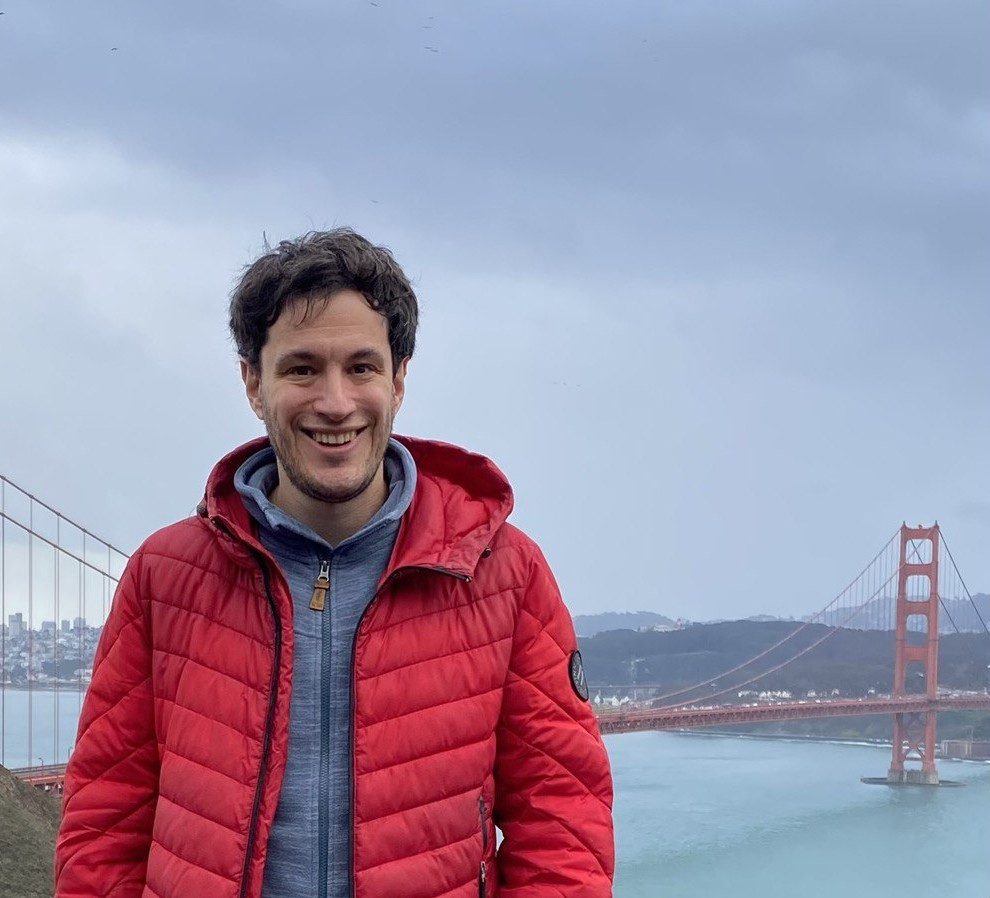# Oleg Butkovsky• Address: Mohrenstrasse 39, 10117 Berlin, Germany.
• Office: Hausvogteiplatz 11, room 0.02
• Phone: +49 30 20372-526.
• e-mail: oleg.butkovsky (at) wias (dash) berlin (dot) de.
• My CV and research statement.
• Most of my papers are available on arXiv.

I am a researcher in the Stochastic Algorithms and Nonparametric Statistics research group at the Weierstrass Institute for Applied Analysis and Stochastics.
Previously, I was a postdoc at Technion, Israel, where I worked with Leonid Mytnik, and at MSRI, California.
I got a PhD in Mathematics at Department of Mathematics of Moscow State University where I was working with Alexander Bulinski and Alexander Veretennikov.

I study how random noise changes the behavior of a complex deterministic system. More precisely, I investigate regularization–by–noise and stabilization–by–noise for ODEs and PDEs, ergodicity and stability for finite and infinite–dimensional Markov processes (stochastic PDEs, stochastic delay equations) as well as the long–term behavior of non–Markov processes (stochastic differential equations driven by fractional Brownian motion, McKean–Vlasov processes). I am also interested in construction of theoretically justified numerical algorithms related to the processes mentioned above.

Events:

## Preprints and Publications

### Regularization by noise for SDEs and SPDEs

1. O. Butkovsky, L. Mytnik (2019). Regularization by noise and flows of solutions for a stochastic heat equation. Annals of Probability, 47(1), 165–212.
2. S. Athreya, O. Butkovsky, L. Mytnik (2020). Strong existence and uniqueness for stable stochastic differential equations with distributional drift. Annals of Probability, 48(1), 178–210.
3. O. Butkovsky, K. Dareiotis, M. Gerencsér (2020). Approximation of SDEs: a stochastic sewing approach. arXiv:1909.07961, to appear in Probability Theory and Related Fields.
4. S. Athreya, O. Butkovsky, K. Lê, L. Mytnik (2020). Well-posedness of stochastic heat equation with distributional drift and skew stochastic heat equation. arXiv:2011.13498.

### Stabilization by noise and ergodicity

1. O. Butkovsky, A.Yu. Veretennikov (2013). On asymptotics for Wasserstein coupling of a Markov chain. Stochastic Processes and their Applications, 123(9), 3518–3541.
2. O. Butkovsky (2014). Subgeometric rates of convergence of Markov processes in the Wasserstein metric. Annals of Applied Probability, 24(2), 526–552.
3. O. Butkovsky, M. Scheutzow (2017). Invariant measures for stochastic functional differential equations. Electronic Journal of Probability, 22(98), 1–23.
4. O. Butkovsky, F. Wunderlich (2019). Asymptotic strong Feller property and local weak irreducibility via generalized couplings. arXiv preprint arXiv:1912.06121.
5. O. Butkovsky, A.Kulik, M. Scheutzow (2020). Generalized couplings and ergodic rates for SPDEs and other Markov models. Annals of Applied Probability, 30(1), 1–39.
6. O. Butkovsky, M. Scheutzow (2020). Couplings via comparison principle and exponential ergodicity of SPDEs in the hypoelliptic setting. Communications in Mathematical Physics, 379(3), 1001–1034.

### McKean–Vlasov equations

1. O. Butkovsky (2012) On the convergence of nonlinear Markov chains. Doklady Mathematics, 86(3), 824–826.
2. O. Butkovsky (2014) On ergodic properties of stochastic McKean–Vlasov equations. Theory of Probability and Its Applications, 58(4), 661–674.